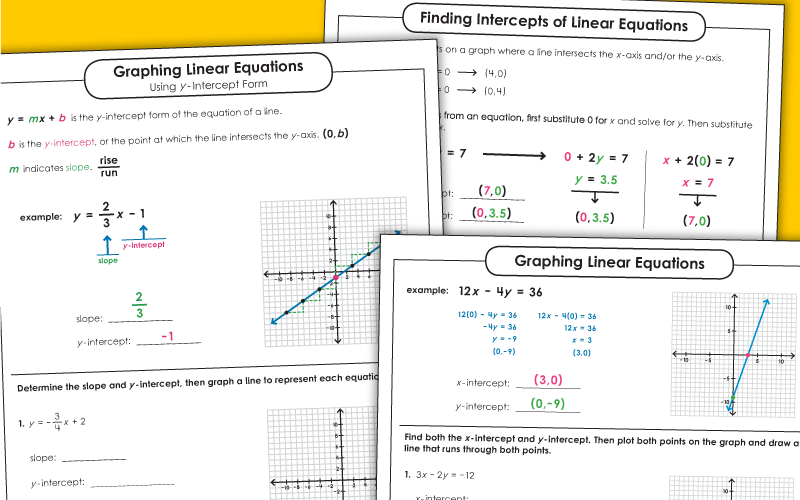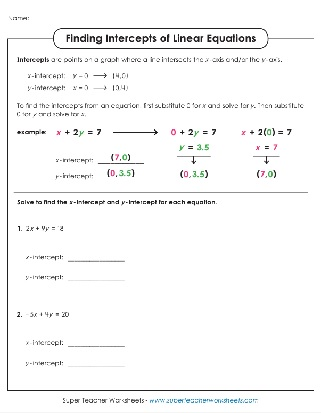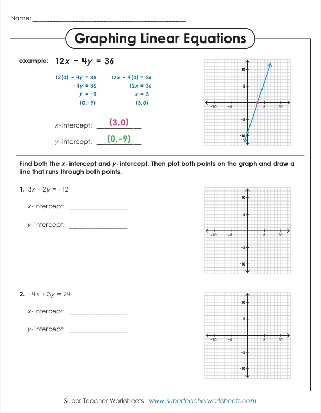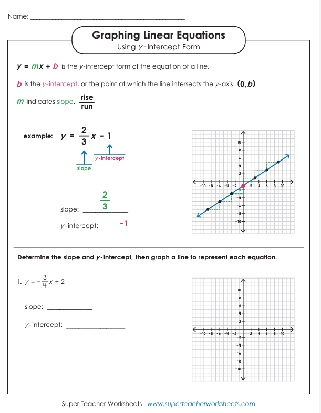# Linear Equations

On these printable worksheets, students will practice solving, finding intercepts, and graphing linear equations.Solve to find the x- and y-intercepts. There are 6 problems to complete on this double-sided worksheet.
Solve to find the x-intercept and y-intercept for each equation. Then plot both points and draw a line to graph the equations.
For each problem, students will define the slope and y-intercept. Then they graph a line to show the equation.
Algebra & Pre-Algebra

STW has a variety of algebra and pre-algebra topics, including: evaluating algebraic expressions, algebraic equations (single-step), algebraic equations (two-step), dependent & independent variables, and inequalities.

## Sample Worksheet ImagesMy Account
Site Information